## Astronomers have recently observed stars orbiting at very high speeds around an unknown object near the center of our galaxy. For stars orbi

Question

Astronomers have recently observed stars orbiting at very high speeds around an unknown object near the center of our galaxy. For stars orbiting at distances of about 1014 m from the object, the orbital velocities are about 106 m/s. Assume the orbits are circular, and estimate the mass of the object, in units of the mass of the sun (MSun = 2×1030 kg). If the object was a tightly packed cluster of normal stars, it should be a very bright source of light. Since no visible light is detected coming from it, it is instead believed to be a supermassive black hole.

in progress 0
5 months 2021-08-12T14:23:06+00:00 1 Answers 8 views 0

The mass of the object is 745000 units of the sun

Explanation:

We know that the centripetal force with which the stars orbit the object is represented as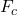=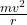and this centripetal force is also proportional to=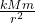where

m is the mass of the stars

M is the mass of the object

v is the velocity of the stars = 10^6 m/s

r is the distance between the stars and the object = 10^14 m

k is the gravitational constant = 6.67 × 10^-11 m^3 kg^-1 s^-2

We can equate the two centripetal force equations to give=which reduces to=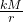and then finally

M =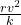substituting values, we have

M =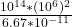= 1.49 x 10^36 kg

If the mass of the sun is 2 x 10^30 kg

then, the mass of the the object in units of the mass of the sun is

==> (1.49 x 10^36)/(2 x 10^30) = 745000 units of sun